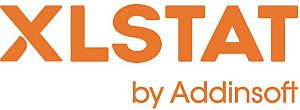# XLSTAT - Agglomerative Hierarchical Clustering (AHC)

## Advantages of Agglomerative Hierarchical Clustering

Agglomerative Hierarchical Clustering (AHC) is a classification method which has the following advantages:

• It works from the dissimilarities between the objects to be grouped together. A type of dissimilarity can be suited to the subject studied and the nature of the data.
• One of the results is the dendrogram which shows the progressive grouping of the data. It is then possible to gain an idea of a suitable number of classes into which the data can be grouped.

## Principle of Agglomerative Hierarchical Clustering

Agglomerative Hierarchical Clustering (AHC) is an iterative classification method whose principle is simple.

1. The process starts by calculating the dissimilarity between the N objects.
2. Then two objects which when clustered together minimize a given agglomeration criterion, are clustered together thus creating a class comprising these two objects.
3. Then the dissimilarity between this class and the N-2 other objects is calculated using the agglomeration criterion. The two objects or classes of objects whose clustering together minimizes the agglomeration criterion are then clustered together.

This process continues until all the objects have been clustered.

These successive clustering operations produce a binary clustering tree (dendrogram), whose root is the class that contains all the observations. This dendrogram represents a hierarchy of partitions. It is then possible to choose a partition by truncating the tree at a given level, the level depending upon either user-defined constraints (the user knows how many classes are to be obtained) or more objective criteria.

## Agglomerative Hierarchical Clustering aggregation methods

XLSTAT proposes several aggregation methods:

• Ward's method (iniertia)
• Ward's method (variance)
• Unweighted pair-group average
• Weighted pair-group average

## Proximities used in Agglomerative Hierarchical Clustering

The proximity between two objects is measured by measuring at what point they are similar (similarity) or dissimilar (dissimilarity). If the user chooses a similarity, XLSTAT converts it into a dissimilarity as the AHC algorithm uses dissimilarities. The conversion for each object pair consists in taking the maximum similarity for all pairs and subtracting from this the similarity of the pair in question.

XLSTAT proposes several similarities/dissimilarities that are suitable for a particular type of data:

 Similarity Dissimilarity Quantitative data Pearson's coefficient of correlation Spearman's coefficient of rank correlation Kendall's coefficient of rank correlation Inertia Covariance (n) Covariance (n-1) Percent agreement Euclidean distance Chi-square distance Manhattan distance Pearson's dissimilarity Spearman's dissimilarity Kendall's dissimilarity Percent disagreement Binary data (0/1) Jaccards coefficient Dice coefficient Sokal & Sneath coefficient (2) Rogers & Tanimoto coefficient Simple matching coefficient Indice de Sokal & Sneath coefficient (1) Phi coefficient Ochiais coefficient Kulczinskis coefficient Percent agreement Jaccards coefficient Dice coefficient Sokal & Sneath coefficient (2) Rogers & Tanimoto coefficient Simple matching coefficient Indice de Sokal & Sneath coefficient (1) Phi coefficient Ochiais coefficient Kulczinskis coefficient Percent agreement

Note: For non-binary categorical variables, it is preferable to first perform a Multiple Correspondence Analysis (MCA) and to consider the coordinates of the observations on the factorial axes as new variables.

## Results provided by XLSTAT for Agglomerative Hierarchical Clustering

• Node statistics:
This node statistics table shows the data for the successive nodes in the dendrogram. The first node has an index which is the number of objects increased by 1. Hence it is easy to see at any time if an object or group of objects is clustered with another object or group of objects at the level of a new node in the dendrogram.
• Levels bar chart:
The level bar chart table displays the statistics for dendrogram nodes.
• Dendrograms:
The full dendrogram displays the progressive clustering of objects. If truncation has been requested, a broken line marks the level the truncation has been carried out. The truncated dendrogram shows the classes after truncation.
• Class centroids:
This class centroids table shows the class centroids for the various descriptors.
• Distance between the class centroids:
The distance between the class centroids table shows the Euclidean distances between the class centroids for the various descriptors.
• Central objects:
The central objects table shows the coordinates of the nearest object to the centroid for each class.
• Distance between the central objects:
This distance between the central objects table shows the Euclidean distances between the class central objects for the various descriptors.
• Results by class:
The descriptive statistics for the classes (number of objects, sum of weights, within-class variance, minimum distance to the centroid, maximum distance to the centroid, mean distance to the centroid) are displayed in the first part of the table. The second part shows the objects.
• Results by object:
The results by object table shows the assignment class for each object in the initial object order.This analysis is available in the XLStat-Basic addin for Microsoft ExcelKovach Computing Services (KCS) was founded in 1993 by Dr. Warren Kovach. The company specializes in the development and marketing of inexpensive and easy-to-use statistical software for scientists, as well as in data analysis consulting.

### Get in Touch

• Email:
sales@kovcomp.com